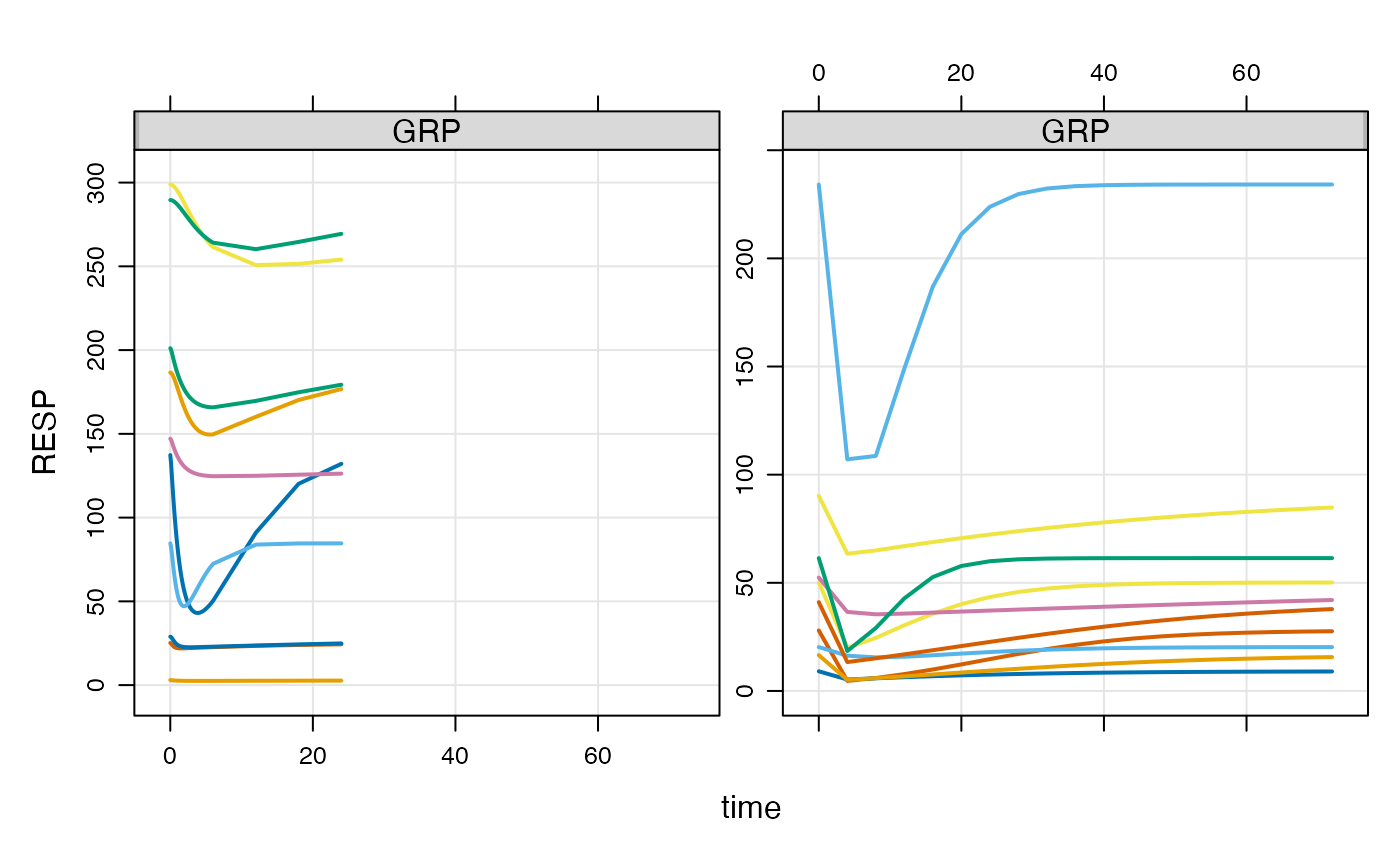This function also allows you to assign different designs to different groups or individuals in a population.

## Usage

design(x, deslist = list(), descol = character(0), ...)

## Arguments

x

model object

deslist

a list of tgrid or tgrids objects or numeric vector to be used in place of ...

descol

the idata column name (character) for design assignment

...

not used

## Details

This setup requires the use of an idata_set, with individual-level data passed in one ID per row. For each ID, specify a grouping variable in idata (descol). For each unique value of the grouping variable, make one tgrid object and pass them in order as ... or form them into a list and pass as deslist.

You must assign the idata_set before assigning the designs in the command chain (see the example below).

## Examples


peak <- tgrid(0,6,0.1)
sparse <- tgrid(0,24,6)

des1 <- c(peak,sparse)
des2 <- tgrid(0,72,4)

data <- expand.ev(ID = 1:10, amt=c(100,300))
data$GRP <- data$amt/100

idata <- data[,c("ID", "amt")]

mod <- mrgsolve::house()

mod %>%
omat(dmat(1,1,1,1)) %>%
carry_out(GRP) %>%
idata_set(idata) %>%
design(list(des1, des2),"amt") %>%
data_set(data) %>%
mrgsim() %>%
plot(RESP~time|GRP)